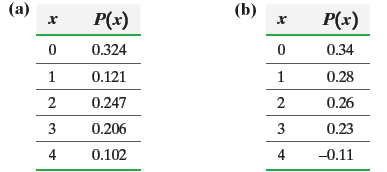×
Get Full Access to Statistics: Informed Decisions Using Data - 5 Edition - Chapter 6 - Problem 2
Get Full Access to Statistics: Informed Decisions Using Data - 5 Edition - Chapter 6 - Problem 2

×

# ?Determine whether the distribution is a discrete probability distribution. If not, state why.ISBN: 9780134133539 240

## Solution for problem 2 Chapter 6

Statistics: Informed Decisions Using Data | 5th Edition

• Textbook Solutions
• 2901 Step-by-step solutions solved by professors and subject experts
• Get 24/7 help from StudySoup virtual teaching assistantsStatistics: Informed Decisions Using Data | 5th Edition

4 5 1 426 Reviews
23
1
Problem 2

Determine whether the distribution is a discrete probability distribution. If not, state why.Step-by-Step Solution:

Step 1 of 5) Determine whether the distribution is a discrete probability distribution. If not, state why. Test Hypotheses Regarding Two Population Standard Deviations Now that we know the approximate sampling distribution of s2 1 s2 2 and how to find critical values in the F-distribution, we can introduce a procedure that can be used to test hypotheses regarding two population standard deviations (or variances). Test Hypotheses Regarding Two Population Standard Deviations To test hypotheses regarding two population standard deviations, s1 and s2, use the following steps, provided that 1. the samples are obtained using simple random sampling or through a randomized experiment. 2. the sample data are independent. 3. the populations from which the samples are drawn are normally distributed. Step 1 Determine the null and alternative hypotheses.

Step 2 of 3

Step 3 of 3

## Discover and learn what students are asking

Statistics: Informed Decisions Using Data : The Normal Approximation to the Binomial Probability Distribution
?In Problems 5–14, a discrete random variable is given. Assume the probability of the random variable will be approximated using the normal distributio

Statistics: Informed Decisions Using Data : Estimating the Value of a Parameter
?For what proportion of samples will a 90% confidence interval for a population mean not capture the true population mean?

Statistics: Informed Decisions Using Data : Tests for Independence and the Homogeneity of Proportions
?Dropping a Course A survey was conducted at a community college of 50 randomly selected students who dropped a course in the current semester to learn

Unlock Textbook Solution